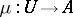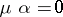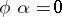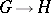# Normal monomorphism

(diff) ← Older revision | Latest revision (diff) | Newer revision → (diff)
A morphism having the characteristic property of an imbedding of a group (ring) into a group (ring) as a normal subgroup (ideal). Letbe a category with zero morphisms. A morphismis called a normal monomorphism if every morphismfor which it always follows from,, that, can be uniquely represented in the form. The kernel of any morphism (cf. Kernel of a morphism in a category) is a normal monomorphism. The converse is not true, in general; however, if cokernels (cf. Cokernel) of morphisms exist in, then every normal monomorphism turns out to be the kernel of its cokernel. In an Abelian category every monomorphism is normal. The concept of a normal monomorphism is dual to that of a normal epimorphism.
The above definition is not entirely standard: many authors would define a normal monomorphism to be a morphism which occurs as a kernel. (The term normal subobject is also in use, for an isomorphism class of normal monomorphisms.) In categories without zero morphisms, a substitute for normal monomorphisms is provided by regular monomorphisms, which are those morphisms which occur as equalizers (cf. Kernel of a morphism in a category). Every normal monomorphism is regular, but not conversely: in the category of all groups every injective homomorphism is a regular monomorphism, but a normal monomorphismis an isomorphism ofonto a normal subgroup of. However, in an additive category the concepts of normal monomorphism and regular monomorphism coincide.